# Selina Concise Mathematics Class 8 ICSE Solutions Chapter 8 Profit, Loss and Discount

## Selina Concise Mathematics Class 8 ICSE Solutions Chapter 8 Profit, Loss and Discount

Selina Publishers Concise Mathematics Class 8 ICSE Solutions Chapter 8 Profit, Loss and Discount

### Profit, Loss and Discount Exercise 8A – Selina Concise Mathematics Class 8 ICSE Solutions

Question 1.
Megha bought 10 note-books for Rs.40 and sold them at Rs.4.75 per note-book. Find, her gain percent.
Solution: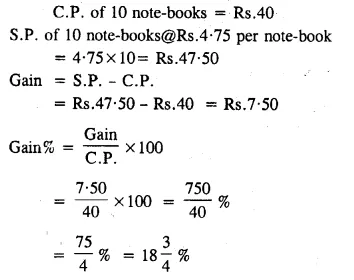Question 2.
A fruit-seller buys oranges at 4 for Rs.3 and sells them at 3 for Rs.4 Find his profit percent.
Solution: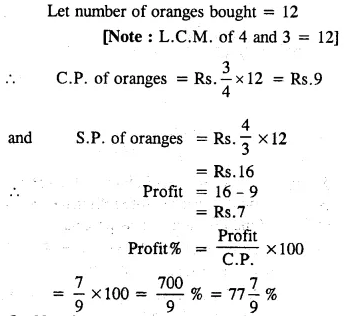Question 3.
A man buys a certain number of articles at 15 for Rs. 112.50 and sells them at 12 for Rs.108. Find ;
(i) his gain as percent;
(ii) the number of articles sold to make a profit of Rs.75.
Solution: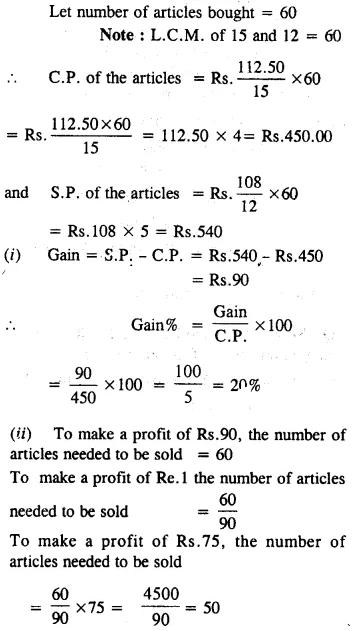Question 4.
A boy buys an old bicycle for Rs. 162 and spends Rs. 18 on its repairs before selling the bicycles for Rs. 207. Find his gain or loss percent.
Solution: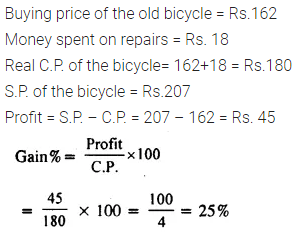Question 5.
An article is bought from Jaipur for Rs. 4,800 and is sold in Delhi for Rs. 5,820. If Rs. 1,200 is spent on its transportations, etc. ; find he loss or the gain as percent.
Solution: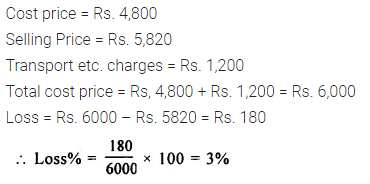Question 6.
Mohit sold a T.V. for Rs. 3,600 ; gaining one-sixth of its selling price. Find :
(i) the gain
(ii) the cost price of the T.V.
(iii) the gain percent.
Solution:Question 7.
By selling a certain number of goods for Rs. 5,500; a shopkeeper loses equal to one-tenth of their selling price. Find :
(i) the loss incured
(ii) the cost price of the goods
(iii) the loss as percent.
Solution: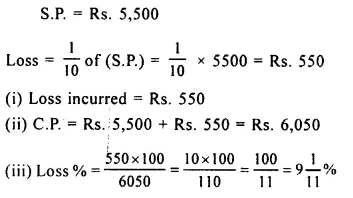Question 8.
The selling price of a sofa-set is $$\frac { 4 }{ 5 }$$ times of its cost price. Find the gain or the loss as percent.
Solution:Question 9.
The cost price of an article is $$\frac { 4 }{ 5 }$$ times of its selling price. Find the loss or the gain as percent.
Solution: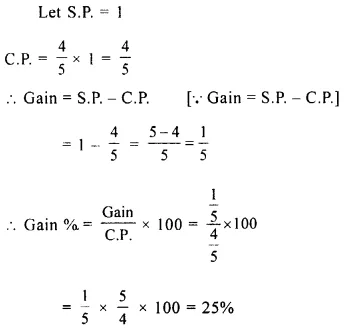Question 10.
A shopkeeper sells his goods at 80% of their cost price. Find the percent gain or loses?
Solution:Question 11.
The cost price of an article is 90% of its selling price. What is the profit or the loss as percent?
Solution:Question 12.
The cost price of an article is 30 percent less than its selling price. Find, the profit or loss as percent.
Solution: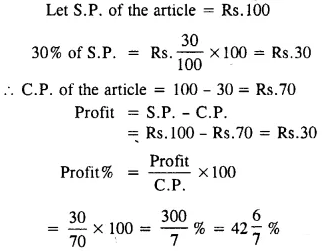Question 13.
A shop-keeper bought 300 eggs at 80 paisa each. 30 eggs were broken in transaction and then he sold the remaining eggs at one rupee each. Find, his gain or loss as percent.
Solution: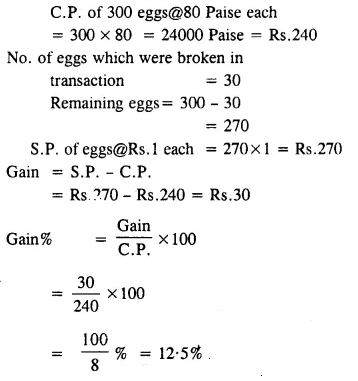Question 14.
A man sold his bicycle for Rs.405 losing one-tenth of its cost price, find :
(i) its cc price;
(ii) the loss percent.
Solution: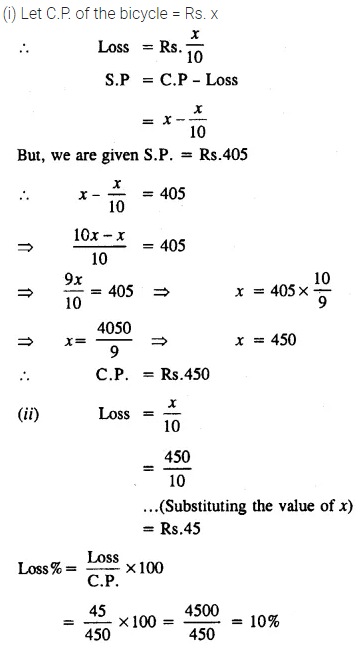Question 15.
A man sold a radio-set for Rs.250 and gained one-ninth of its cost price. Find ;
(i) its cost price;
(ii) the profit percent.
Solution: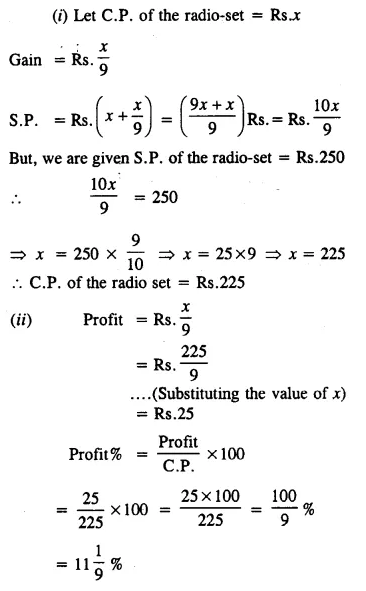### Profit, Loss and Discount Exercise 8B – Selina Concise Mathematics Class 8 ICSE Solutions

Question 1.
Find the selling price, if:
(i) C.P. = Rs. 950 and profit = 8%
(ii) C.P. = Rs. 1,300 and loss = 13%
Solution:Question 2.
Find the cost price, if :
(i) S.P. = Rs. 1,680 and profit = 12%
(ii) S.P. = Rs. 1,128 and loss = 6%
Solution: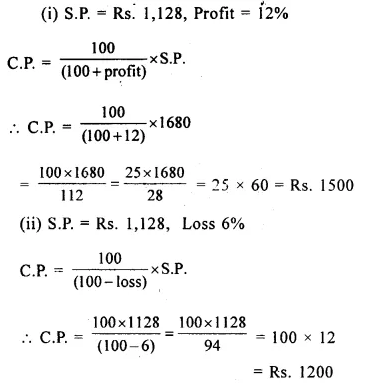Question 3.
By selling an article for Rs.900; a man gains 20%. Find his cost price and the gain.
Solution: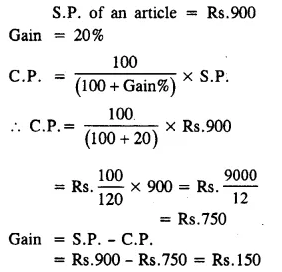Question 4.
By selling an article for Rs.704; a person loses 12%. Find his cost price and the loss
Solution: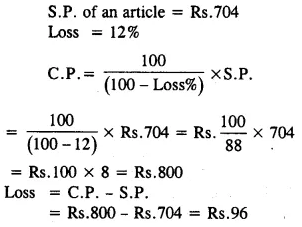Question 5.
Find the selling price, if :
(i) C.P. = Rs.352; overheads = Rs.28 and profit = 20
(ii) C.P. = Rs.576; overheads = Rs.44 and loss = 16%
Solution: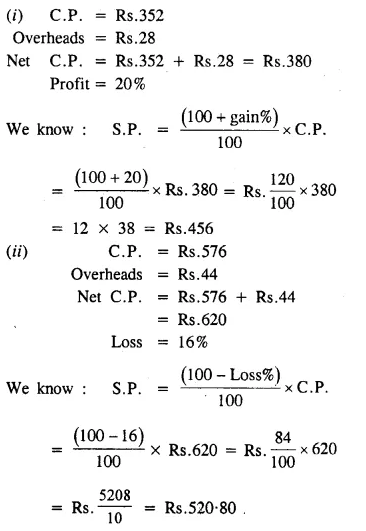Question 6.
If John sells his bicycle for Rs. 637, he will suffer a loss of 9%. For how much should it be sold, if he desires a profit of 5%?
Solution:Question 7.
A man sells a radio-set for Rs.605 and gains 10%. At what price should he sell another radio of the same kind, in order to gain 16%?
Solution:Question 8.
By selling a sofa-set for Rs.2,500; the shopkeeper loses 20%. Find his loss percent or profit percent ; if he sells the same sofa-set for Rs.3150.
Solution: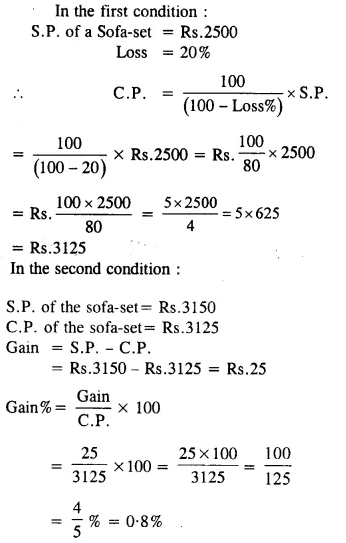Question 9.
Mr. Sinha sold two tape-recorders for Rs.990 each; gaining 10% on one and losing 10% on the other. Find his total loss or gain as percent on the whole transaction.
Solution: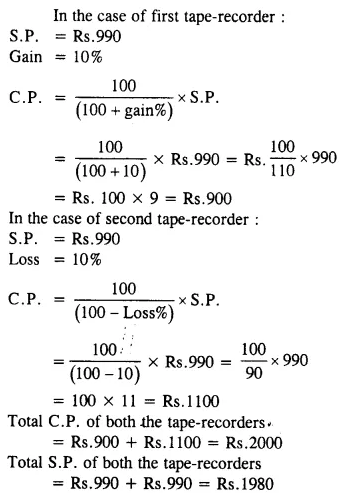Question 10.
A tape-recorder is sold for Rs. 2,760 at a gain of 15% and a C.D. player is sold for Rs. 3,240 at a loss of 10% Find :
(i) the C.P. of the tape-recorder
(ii) the C.P. of the C.D. player.
(iii) the total C.P. of both.
(iv) the total S.P. of both
(v) the gain % or the loss % on the whole
Solution:Question 11.
Rajesh sold his scooter to Rahim at 8% loss and Rahim, in turn, sold the same scooter to Prem at 5% gain. If Prem paid Rs. 14,490 for the scooter ; find :
(i) the S.P. and the C.P. of the scooter for Rahim
(ii) the S.P. and the C.P. of the scooter for Rajesh
Solution:Question 12.
John sold an article to Peter at 20% profit and Peter sold it to Mohan at 5% loss. If Mohan paid Rs.912 for the article; find how much did John pay for it ?
Solution:### Profit, Loss and Discount Exercise 8C – Selina Concise Mathematics Class 8 ICSE Solutions

Question 1.
A stationer buys pens at 5 for Rs.28 and sells them at a profit of 25 %. How much should a customer pay; if he buys
(i) only one pen ;
(ii) three pens ?
Solution: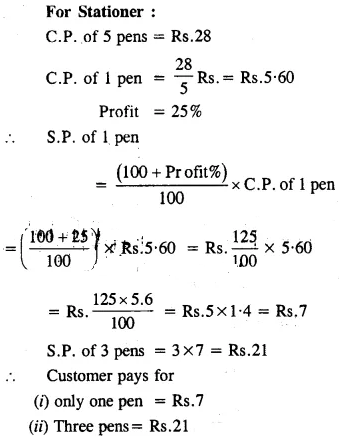Question 2.
A fruit-seller sells 4 oranges for Rs. 3, gaining 50%. Find :
(i) C.P. of 4 oranges,
(ii) C.P. of one orange.
(iii) S.P. of one orange.
(iv) profit made by selling one orange.
(v) number of oranges brought and sold in order to gain Rs. 24.
Solution: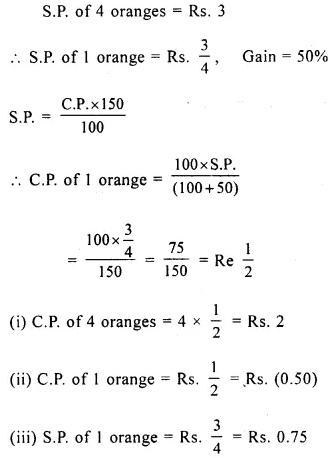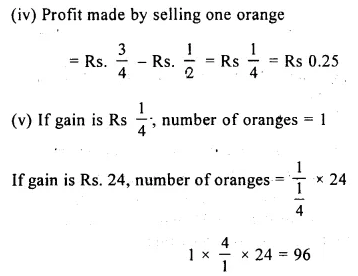Question 3.
A man sells 12 articles for Rs. 80 gaining 33$$\frac { 1 }{ 3 }$$ %. Find the number of articles bought by the man for Rs. 90.
Solution: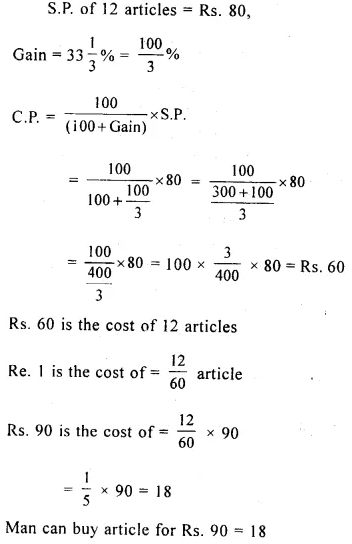Question 4.
The cost price of 20 articles is same as the selling price of 16 articles. Find the gain percent.
Solution:Question 5.
The selling price of 15 articles is equal to the cost price of 12 articles. Find the gain or loss as percent.
Solution:Question 6.
By selling 8 pens, Shyam loses equal to the cost price of 2 pens. Find his loss percent.
Solution:Question 7.
A shop-keeper bought rice worth Rs.4,500. He sold one-third of it at 10% profit.
If he desires a profit of 12% on the whole ; find :
(i) the selling price of the rest of the rice ;
(ii) the percentage profit on the rest of the rice.
Solution:Question 8.
Mohan bought a certain number of note-books for Rs.600. He sold $$\frac { 1 }{ 4 }$$ of them at 5 percent loss. At what price should he sell the remaining note-books so as to gain 10% on the whole ?
Solution:Question 9.
Raju sells a watch at 5% profit. Had he sold it for Rs.24 more ; he would have gained 11%. Find the cost price of the watch.
Solution: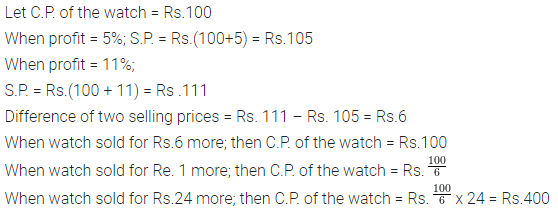Question 10.
A man sold a bicycle at 5% profit. If the cost had been 30% less and the selling price Rs.63 less, he would have made a profit of 30%. What is the cost price of the bicycle?
Solution:Question 11.
Renu sold an article at a loss of 8 percent. Had she bought it at 10% less and sold for Rs.36 more; she would have gained 20%. Find the cost price of the article.
Solution: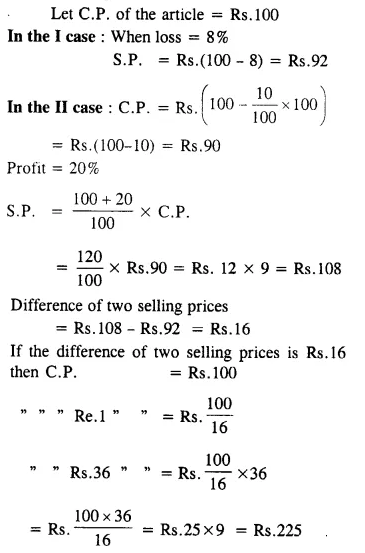### Profit, Loss and Discount Exercise 8D – Selina Concise Mathematics Class 8 ICSE Solutions

Question 1.
An article is marked for Rs. 1,300 and is sold for Rs. 1,144 ; find the discount percent.
Solution: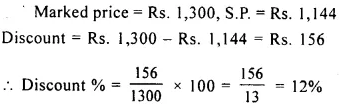Question 2.
The marked price of a dinning table is Rs. 23,600 and is available at a discount of 8%. Find its selling price.
Solution: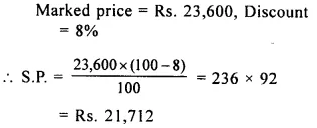Question 3.
A wrist-watch is available at a discount of 9%. If the list-price of the watch is Rs. 1,400 ; find the discount given and the selling price of the watch.
Solution:Question 4.
A shopkeeper sells an article for Rs. 248.50 after allowing a discount of 10%. Find the list price of the article.
Solution: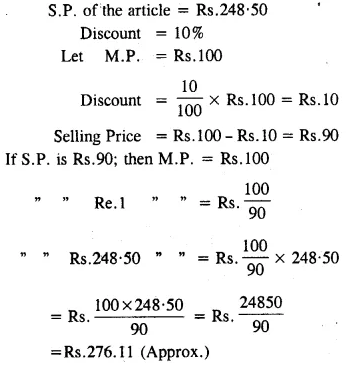Question 5.
A shop-keeper buys an article for Rs.450. He marks it at 20% above the cost price. Find :
(i) the marked price of the article.
(ii) the selling price, if he sells the articles at 10 percent discount.
(iii) the percentage discount given by him, if he sells the article for Rs.496.80
Solution: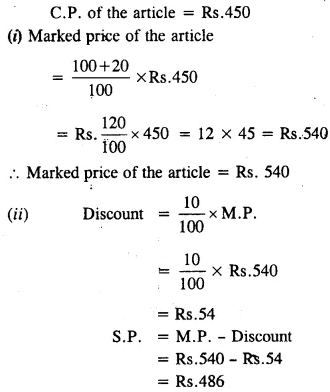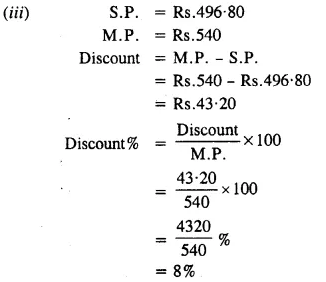Question 6.
The list price of an article is Rs.800 and is available at a discount of 15 percent. Find :
(i) selling price of the article ;
(ii) cost price of the article, if a profit of 13$$\frac { 1 }{ 3 }$$ % is made on selling it.
Solution:Question 7.
An article is marked at Rs. 2,250. By selling it at a discount of 12%, the dealer makes a profit of 10%. Find :
(i) the selling price of the article.
(ii) the cost price of the article for the dealer.
Solution:Question 8.
By selling an article at 20% discount, a shopkeeper gains 25%. If the selling price of the article is Rs. 1,440 ; find :
(i) the marked price of the article.
(ii) the cost price of the article.
Solution: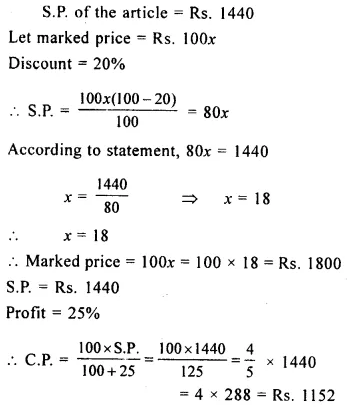Question 9.
A shop-keeper marks his goods at 30 percent above the cost price and then gives a discount of 10 percent. Find his gain percent.
Solution: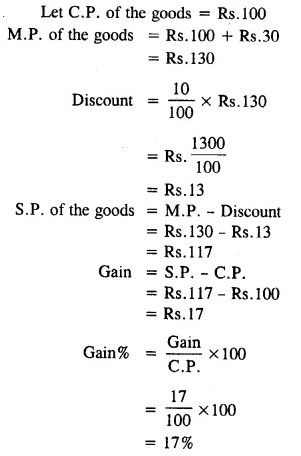Question 10.
A ready-made garments shop in Delhi, allows 20 percent discount on its garments and still makes a profit of 20 percent. Find the marked price of a dress which is bought by the shop-keeper for Rs.400.
Solution: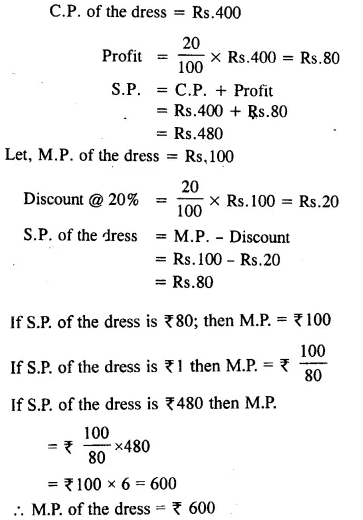Question 11.
At 12% discount, the selling price of a pen is Rs. 13.20. Find its marked price. Also, find the new selling price of the pen, if it is sold at 5% discount.
Solution:Question 12.
The cost price of an article is Rs. 2,400 and it is marked at 25% above the cost price. Find the profit and the profit percent, if the article is sold at 15% discount.
Solution:Question 13.
Thirty articles are bought at Rs. 450 each. If one-third of these articles be sold at 6% loss; at what price must each of the remaining articles be sold in order to make a profit of 10% on the whole?
Solution: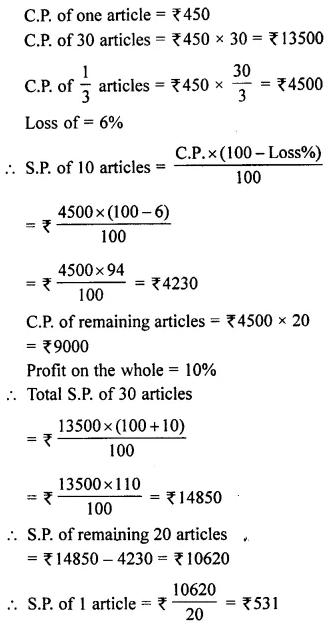Question 14.
The cost price of an article is 25% below the marked price. If the article is available at 15% discount and its cost price is Rs. 2,400; find:
(i) Its marked price
(ii) its selling price
(iii) the profit percent.
Solution:Question 15.
Find a single discount (as percent) equivalent to following successive discounts:
(i) 20% and 12%
(ii) 10%, 20% and 20%
(iii) 20%, 10% and 5%
Solution: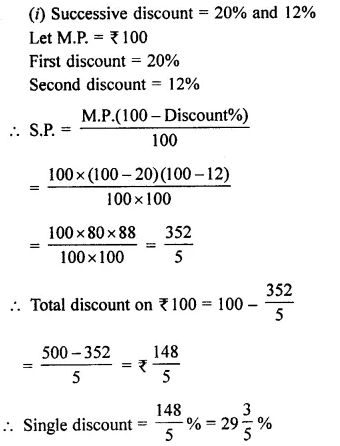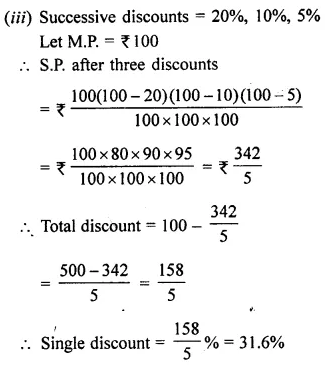Question 16.
Find the single discount (as percent) equivalent to successive discounts of:
(i) 80% and 80%
(ii) 60% and 60%
(iii) 60% and 80%
Solution:### Profit, Loss and Discount Exercise 8E – Selina Concise Mathematics Class 8 ICSE Solutions

Question 1.
Rajat purchases a wrist-watch costing Rs. 540. The rate of Sales Tax is 8%. Find the total amount paid by Rajat for the watch.
Solution: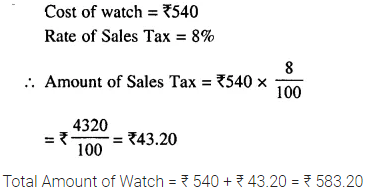Question 2.
Ramesh paid ₹ 345.60 as Sales Tax on a purchase of ₹ 3,840. Find the rate of Sales Tax.
Solution: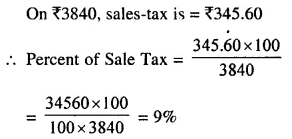Question 3.
The price of a washing machine, inclusive of sales tax is ₹ 13,530/-. If the Sales Tax is 10%, find its basic (cost) price.
Solution: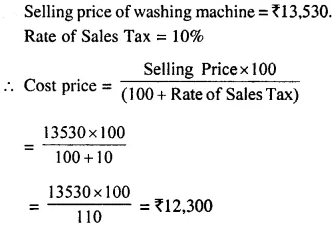Question 4.
Sarita purchases biscuits costing ₹ 158 on which the rate of Sales Tax is 6%. She also purchases some cosmetic goods costing ₹ 354 on which rate of Sales Tax is 9%. Find the total amount to be paid by Sarita.
Solution:Question 5.
The price of a T.V. set inclusive of sales tax of 9% is ₹ 13,407. Find its marked price. If Sales Tax is increased to 13%, how much more does the customer has to pay for the T.V. ?
Solution: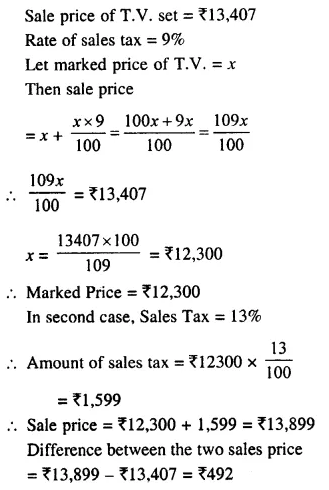Question 6.
The price of an article is ₹ 8,250 which includes Sales Tax at 10%. Find how much more or less does a customer pay for the article, if the Sales Tax on the article:
(i) increases to 15%
(ii) decreases to 6%
(iii) increases by 2%
(iv) decreases by 3%
Solution: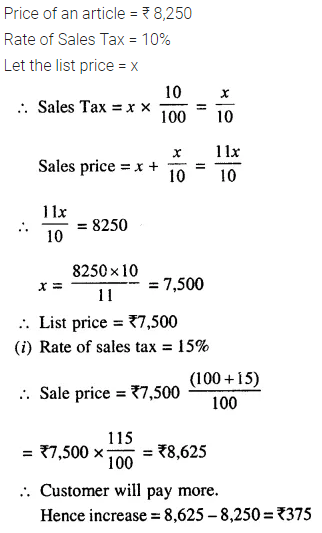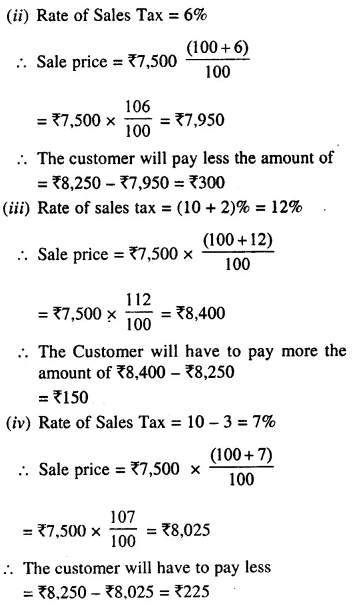Question 7.
A bicycle is available for ₹ 1,664 including Sales Tax. If the list price of the bicycle is ₹ 1,600, find :
(i) the rate of Sales Tax
(ii) the price a customer will pay for the bicycle if the Sales Tax is increased by 6%.
Solution: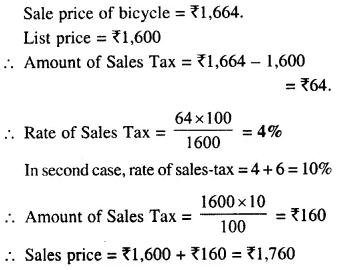Question 8.
When the rate of sale-tax is decreased from 9% to 6% for a coloured T.V. ; Mrs. Geeta will save ₹ 780 in buying this T.V. Find the list price of the T.V.
Solution: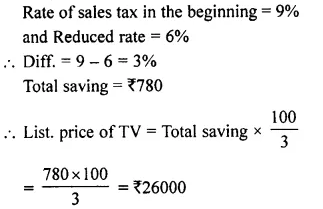Question 9.
A shopkeeper sells an article for ₹ 21,384 including 10% sales-tax. However, the actual rate of sales-tax is 8%. Find the extra profit made by the dealer.
Solution:### Profit, Loss and Discount Exercise 8F – Selina Concise Mathematics Class 8 ICSE Solutions

[In this exercise, all the prices are excluding tax/VAT unless specified]
Question 1.
A shopkeeper buys an article for ₹ 8,000 and sells it for ₹ 10,000. If the rate of tax under VAT is 10%, find :
(i) tax paid by the shopkeeper
(ii) tax charged by the shopkeeper
(iii) VAT paid by the shopkeeper
Solution:Question 2.
A trader buys some goods for ₹ 12,000 and sells them for ₹ 15,000. If the rate of tax under VAT is 12%, find the VAT paid by the trader?
Solution:Question 3.
The marked price of an article is ₹ 7,000 and is available at 20% discount. Manoj buys this article and then sold it at its marked price. If the rate of tax at each state is 10%, find the VAT paid by Manoj.
Solution: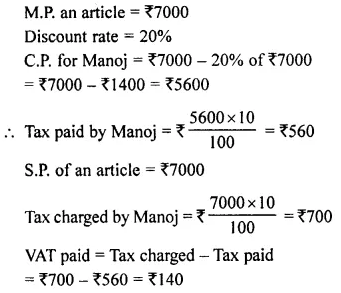Question 4.
A buys some goods for ₹ 4,000 and sold them to B for ₹ 5,000. B sold these goods to C for ₹ 6,000. If the rate of tax (under VAT) at each stage is 5%, find :
(i) VAT paid by A
(ii) VAT paid by B
Solution: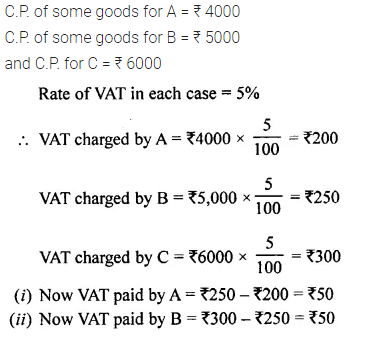Question 5.
A buys an article for ₹ 8,000 and sold it to B at 20% profit. If the rate of tax under VAT is 8%, find :
(i) tax paid by A
(ii) tax charged by A
(iii) VAT paid by A
Solution: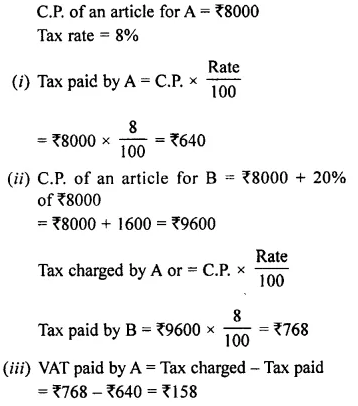Question 6.
A shopkeeper purchases an article for ₹ 12,400 and sells it to a customer for ₹ 17,000. If the tax under VAT is 8%, find the VAT paid by the shopkeeper.
Solution:Question 7.
A purchases an article for ₹ 7,200 and sells it to B for ₹ 9,600. B, in turn, sells the article to C for ₹ 11,000. If the tax (under VAT) is 10%, find the VAT paid by A and B.
Solution: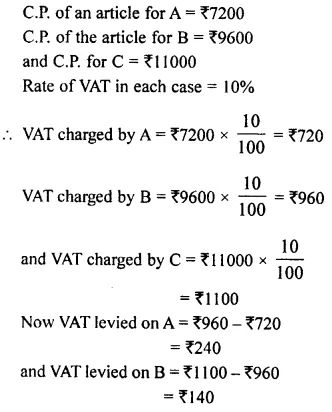Question 8.
A manufacturer buys some goods for ₹ 60,000 and pays 5% tax. He sells these goods for ₹ 80,000 and charges tax at the rate of 12%. Find the VAT paid by the manufacturer.
Solution: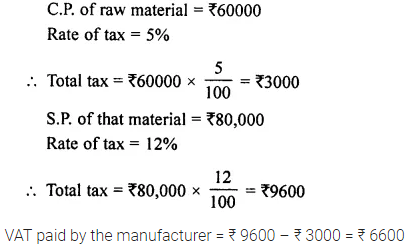Question 9.
The cost of an article is ₹ 6,000 to a distributor, he sells it to a trader for ₹ 7,500 and the trader sells it further to a customer for ₹ 8,000. If the rate of tax under VAT is 8%; find the VAT paid by the:
(i) distributor